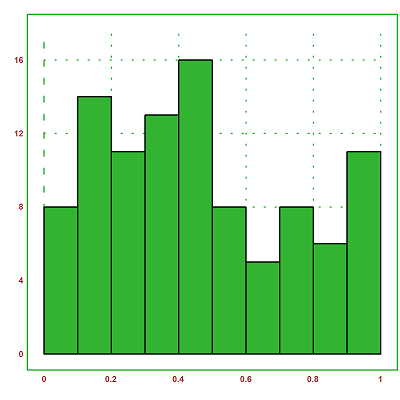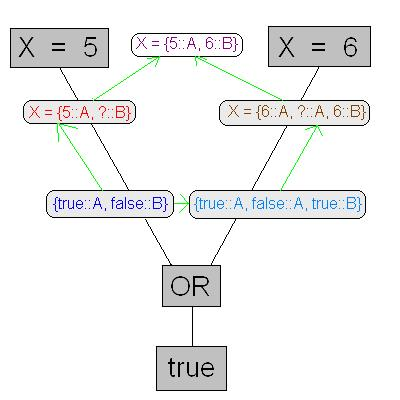# Difference between Variable and Random Variable

The concept of variable is commonly used in the field of physics, engineering and mathematics. Essentially, a variable is defined as the quantity that is dependent on one or more constants. For example, speed is a variable as it depends on the distance covered “d” in time in “t”.

Speed = d/t

Any change in the value of distance or time will result in a change in the value of speed. Variables are represented by various symbols depending on the quantities involved in the equation. They can behave differently based on their properties and definitions.

Random variable on the other hand depends on the entity of the described variable in statistics and probability. They are mostly represented by Capital letters such as X, A, Z, D, K etc. Random variable is related to a state, as shown in the equation below:

P(Y=Z)

Random variables are also used to represent a series of probabilities E(X) in mathematics, where E is the domain of the random variable.

### Instructions

• 1

Variable:

Any quantity possessing changing or variable magnitude is known as a variable in statistics.

It should be noted that variable quantities are required to be calculated to solve complex equations. Speeds, velocity and acceleration are the most commonly used examples of variables.

It is usually represented by a Greek or English letter in the lower case. These symbolic letters are used in the equations that also contain one or more constants. Consider the example of a velocity variable v, which is defined as the speed divided by time. The equation given below is used to compute velocity of a moving object:

V = s/t

Where s is the speed of the moving object and t is the time.

As we can see from the equation, V is directly dependent on time and speed.

Any change in speed and time will result in a change in velocity. Depending on the behaviour and properties, variables can also be categorised. There are two types of variables:

- Independent variables

- Dependent variables

Dependent variables depend on other variables in an equation whereas independent variable depends only on constants.

Image Courtesy: euler.rene-grothmann.de• 2

Random Variable:

Random variables represent an event or a series of events or probabilities.

Random variables can also be categorised as either continuous random variables or discrete random variables. In statistics, dependent random variables and independent random variables are known as response variables and explanatory variables respectively.

Image Courtesy: tau.ac.il•